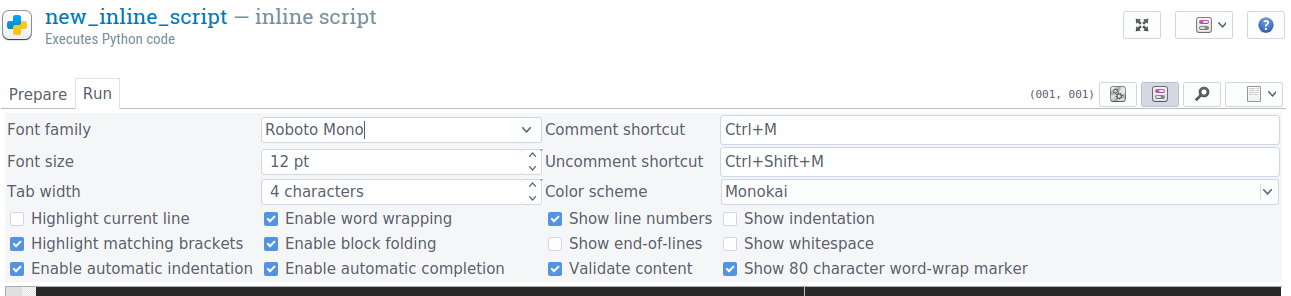#### Howdy, Stranger!

It looks like you're new here. If you want to get involved, click one of these buttons!

#### Categories

Supported by

Hi All,

I am trying to set the canvas "my_target_canvas" to pull up a different canvas depending on what the variable "target_pic" is. I've tried doing this below, but I'm getting an error when it tries to run through the code. All of the sketchpads have been created elsewhere and are being called up by name in the code. Can you see something that might be a problem here or have suggestions for fixing? Of note is that I only just updated to 3.1.2 and had been running on an older version with a similar code except only one possible target, and that code worked in the previous version, so I'm not sure if it's something with the update.

Thanks for the help!
Kristin

`#draw fixation screen canvas
from openexp.canvas import canvas
my_fix_canvas = canvas(exp)

# draw boxes and fixation

my_fix_canvas.prepare()

if var.target_pic == "lightning":
my_target_canvas = canvas(exp)
my_target_canvas.prepare()
else:
if var.target_pic == "star":
my_target_canvas = canvas(exp)
my_target_canvas.prepare()
else:
if var.target_pic == "arrow":
my_target_canvas = canvas(exp)
my_target_canvas.prepare()
else:
if var.target_pic == "flag":
my_target_canvas = canvas(exp)
my_target_canvas.prepare()
else:
if var.target_pic == "8point_star":
my_target_canvas = canvas(exp)
my_target_canvas.prepare()
else:
if var.target_pic == "moon":
my_target_canvas = canvas(exp)
my_target_canvas.prepare()
else:
if var.target_pic == "triangle":
my_target_canvas = canvas(exp)
my_target_canvas.prepare()
else:
if var.target_pic == "heart":
my_target_canvas = canvas(exp)
my_target_canvas.prepare()
`

• ``````#draw fixation screen canvas
from openexp.canvas import canvas
my_fix_canvas = canvas(exp)
#draw boxes and fixation
my_fix_canvas.prepare()

if var.target_pic == "lightning":
my_target_canvas = canvas(exp)
my_target_canvas.prepare()
else:
if var.target_pic == "star":
my_target_canvas = canvas(exp)
my_target_canvas.prepare()
else:
if var.target_pic == "arrow":
my_target_canvas = canvas(exp)
my_target_canvas.prepare()
else:
if var.target_pic == "flag":
my_target_canvas = canvas(exp)
my_target_canvas.prepare()
else:
if var.target_pic == "8point_star":
my_target_canvas = canvas(exp)
my_target_canvas.prepare()
else:
if var.target_pic == "moon":
my_target_canvas = canvas(exp)
my_target_canvas.prepare()
else:
if var.target_pic == "triangle":
my_target_canvas = canvas(exp)
my_target_canvas.prepare()
else:
if var.target_pic == "heart":
my_target_canvas = canvas(exp)
my_target_canvas.prepare()
``````
• Best,
Jarik

• I've tried doing this below, but I'm getting an error when it tries to run through the code.

What's clearly missing in your question is the actual error! Details please.

In any case, the script that you posted is incorrect Python because the indentation is invalid; that is, `else` should always be preceded by just as many tabs as the `if` that it belongs to. You can enable script validation ('Validate content') in OpenSesame to see which lines are incorrect and why (insofar as the validation can detect this, of course):Cheers!
Sebastiaan